# State Space Model for an eletronic circuit

#### Current_Source

Joined Dec 10, 2015
16
Hello!

I am trying to find a space state model for the following circuit. :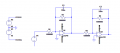My approach was trying to divide the circuit in various blocks and then find the output expression for those blocks.

So i have defined the voltages Vout1,Vout2 has shown in the circuit.

Then i have calculated the ouput expressions for the blocks i have considered and i got: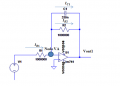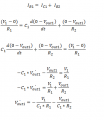For this block i wrote the following set of equations in order to find an expression in order to vout2: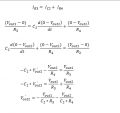So the matrix that represent the space state model of the circuit in the form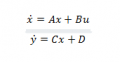So considering the above form i got the following matrix and equations: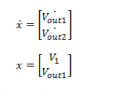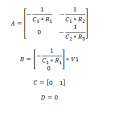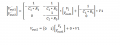Is this correct? Thanks

Last edited: